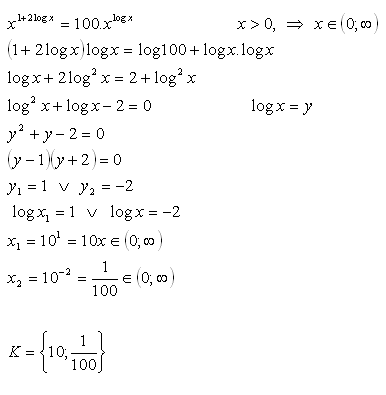sk|cz|

# Logarithmic Exponential Equations

1. Solve:

log3(2x - 7) = 2              2x > 7
Solution:
log3(2x - 7) = 2
log3(2x - 7) = log332
2x – 7 = 32
2x – 7 = 9
2x = 16
2x = 24
x = 4

K = {4}

2. Solve:

log2( 9- 2x) = 3 – x       2x < 9
Solution: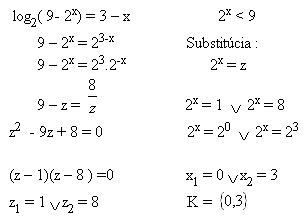3. Solve:

log3( 1 + log3(2x-7)) = 1           2x > 7
Solution:
log3( 1 + log3(2x-7)) = 1
log3( 1+ log3(2x-7)) = log33
1 + log3(2x-7) = 3
log3(2x-7) = 2
log3(2x-7) = log39
2x-7 = 9
2x = 16
2x = 24
x = 4

K = {4}

4. Solve:

log3(3x- 8) = 2 – x      3x > 8
Solution: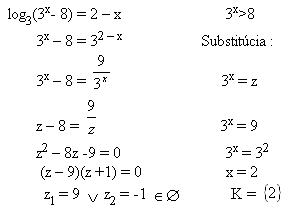5. Solve: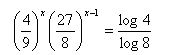Solution: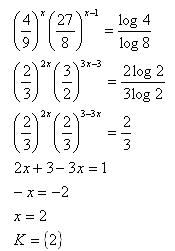5. Solve:Solution:6.Solve: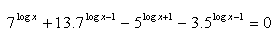Solution: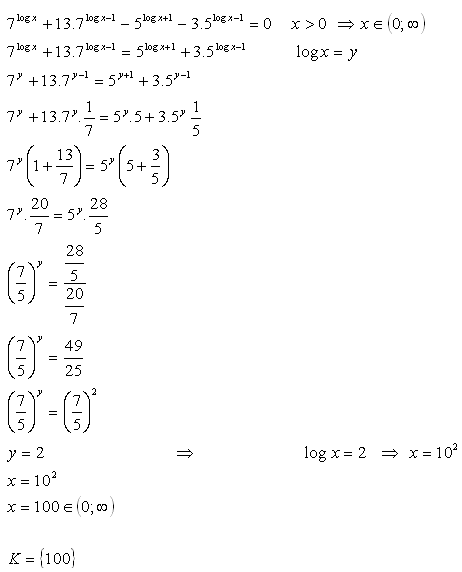7. Solve: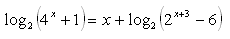Solution: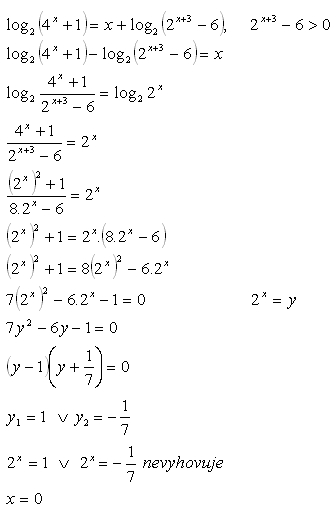8. Solve: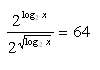Solution: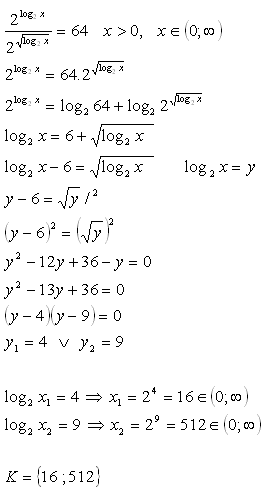9. Solve: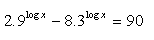Solution: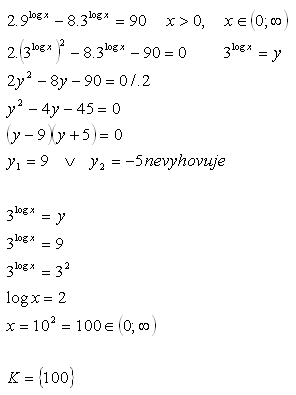10.Solve: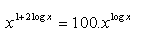Solution: